# 10.4 Polar coordinates: graphs  (Page 7/16)

 Page 7 / 16

Sketch the graph of $\text{\hspace{0.17em}}r=-\theta \text{\hspace{0.17em}}$ over the interval $\text{\hspace{0.17em}}\left[0,4\pi \right].$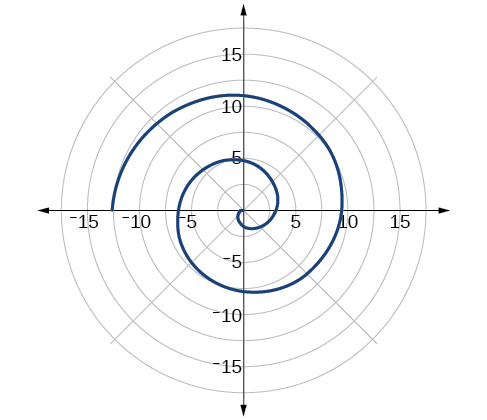## Summary of curves

We have explored a number of seemingly complex polar curves in this section. [link] and [link] summarize the graphs and equations for each of these curves.

Access these online resources for additional instruction and practice with graphs of polar coordinates.

## Key concepts

• It is easier to graph polar equations if we can test the equations for symmetry with respect to the line $\text{\hspace{0.17em}}\theta =\frac{\pi }{2},\text{\hspace{0.17em}}$ the polar axis, or the pole.
• There are three symmetry tests that indicate whether the graph of a polar equation will exhibit symmetry. If an equation fails a symmetry test, the graph may or may not exhibit symmetry. See [link] .
• Polar equations may be graphed by making a table of values for $\text{\hspace{0.17em}}\theta \text{\hspace{0.17em}}$ and $\text{\hspace{0.17em}}r.$
• The maximum value of a polar equation is found by substituting the value $\text{\hspace{0.17em}}\theta \text{\hspace{0.17em}}$ that leads to the maximum value of the trigonometric expression.
• The zeros of a polar equation are found by setting $\text{\hspace{0.17em}}r=0\text{\hspace{0.17em}}$ and solving for $\text{\hspace{0.17em}}\theta .\text{\hspace{0.17em}}$ See [link] .
• Some formulas that produce the graph of a circle in polar coordinates are given by $\text{\hspace{0.17em}}r=a\mathrm{cos}\text{\hspace{0.17em}}\theta \text{\hspace{0.17em}}$ and $\text{\hspace{0.17em}}r=a\mathrm{sin}\text{\hspace{0.17em}}\theta .\text{\hspace{0.17em}}$ See [link] .
• The formulas that produce the graphs of a cardioid are given by $\text{\hspace{0.17em}}r=a±b\mathrm{cos}\text{\hspace{0.17em}}\theta \text{\hspace{0.17em}}$ and $\text{\hspace{0.17em}}r=a±b\mathrm{sin}\text{\hspace{0.17em}}\theta ,\text{\hspace{0.17em}}$ for $\text{\hspace{0.17em}}a>0,\text{\hspace{0.17em}}\text{\hspace{0.17em}}b>0,\text{\hspace{0.17em}}$ and $\text{\hspace{0.17em}}\frac{a}{b}=1.\text{\hspace{0.17em}}$ See [link] .
• The formulas that produce the graphs of a one-loop limaçon are given by $\text{\hspace{0.17em}}r=a±b\mathrm{cos}\text{\hspace{0.17em}}\theta \text{\hspace{0.17em}}$ and $\text{\hspace{0.17em}}r=a±b\mathrm{sin}\text{\hspace{0.17em}}\theta \text{\hspace{0.17em}}$ for $\text{\hspace{0.17em}}1<\frac{a}{b}<2.\text{\hspace{0.17em}}$ See [link] .
• The formulas that produce the graphs of an inner-loop limaçon are given by $\text{\hspace{0.17em}}r=a±b\mathrm{cos}\text{\hspace{0.17em}}\theta \text{\hspace{0.17em}}$ and $\text{\hspace{0.17em}}r=a±b\mathrm{sin}\text{\hspace{0.17em}}\theta \text{\hspace{0.17em}}$ for $\text{\hspace{0.17em}}a>0,\text{\hspace{0.17em}}\text{\hspace{0.17em}}b>0,\text{\hspace{0.17em}}$ and $\text{\hspace{0.17em}}a See [link] .
• The formulas that produce the graphs of a lemniscates are given by $\text{\hspace{0.17em}}{r}^{2}={a}^{2}\mathrm{cos}\text{\hspace{0.17em}}2\theta \text{\hspace{0.17em}}$ and $\text{\hspace{0.17em}}{r}^{2}={a}^{2}\mathrm{sin}\text{\hspace{0.17em}}2\theta ,\text{\hspace{0.17em}}$ where $\text{\hspace{0.17em}}a\ne 0.$ See [link] .
• The formulas that produce the graphs of rose curves are given by $\text{\hspace{0.17em}}r=a\mathrm{cos}\text{\hspace{0.17em}}n\theta \text{\hspace{0.17em}}$ and $\text{\hspace{0.17em}}r=a\mathrm{sin}\text{\hspace{0.17em}}n\theta ,\text{\hspace{0.17em}}$ where $\text{\hspace{0.17em}}a\ne 0;\text{\hspace{0.17em}}$ if $\text{\hspace{0.17em}}n\text{\hspace{0.17em}}$ is even, there are $\text{\hspace{0.17em}}2n\text{\hspace{0.17em}}$ petals, and if $\text{\hspace{0.17em}}n\text{\hspace{0.17em}}$ is odd, there are $\text{\hspace{0.17em}}n\text{\hspace{0.17em}}$ petals. See [link] and [link] .
• The formula that produces the graph of an Archimedes’ spiral is given by $\text{\hspace{0.17em}}r=\theta ,\text{\hspace{0.17em}}\text{\hspace{0.17em}}\theta \ge 0.\text{\hspace{0.17em}}$ See [link] .

## Verbal

Describe the three types of symmetry in polar graphs, and compare them to the symmetry of the Cartesian plane.

Symmetry with respect to the polar axis is similar to symmetry about the $\text{\hspace{0.17em}}x$ -axis, symmetry with respect to the pole is similar to symmetry about the origin, and symmetric with respect to the line $\text{\hspace{0.17em}}\theta =\frac{\pi }{2}\text{\hspace{0.17em}}$ is similar to symmetry about the $\text{\hspace{0.17em}}y$ -axis.

Which of the three types of symmetries for polar graphs correspond to the symmetries with respect to the x -axis, y -axis, and origin?

What are the steps to follow when graphing polar equations?

Test for symmetry; find zeros, intercepts, and maxima; make a table of values. Decide the general type of graph, cardioid, limaçon, lemniscate, etc., then plot points at and sketch the graph.

Describe the shapes of the graphs of cardioids, limaçons, and lemniscates.

What part of the equation determines the shape of the graph of a polar equation?

The shape of the polar graph is determined by whether or not it includes a sine, a cosine, and constants in the equation.

## Graphical

For the following exercises, test the equation for symmetry.

$r=5\mathrm{cos}\text{\hspace{0.17em}}3\theta$

$r=3-3\mathrm{cos}\text{\hspace{0.17em}}\theta$

symmetric with respect to the polar axis

$r=3+2\mathrm{sin}\text{\hspace{0.17em}}\theta$

$r=3\mathrm{sin}\text{\hspace{0.17em}}2\theta$

symmetric with respect to the polar axis, symmetric with respect to the line $\theta =\frac{\pi }{2},$ symmetric with respect to the pole

$r=4$

$r=2\theta$

no symmetry

$r=4\mathrm{cos}\text{\hspace{0.17em}}\frac{\theta }{2}$

$r=\frac{2}{\theta }$

no symmetry

$r=3\sqrt{1-{\mathrm{cos}}^{2}\theta }$

$r=\sqrt{5\mathrm{sin}\text{\hspace{0.17em}}2\theta }$

symmetric with respect to the pole

For the following exercises, graph the polar equation. Identify the name of the shape.

$r=3\mathrm{cos}\text{\hspace{0.17em}}\theta$

$r=4\mathrm{sin}\text{\hspace{0.17em}}\theta$

circle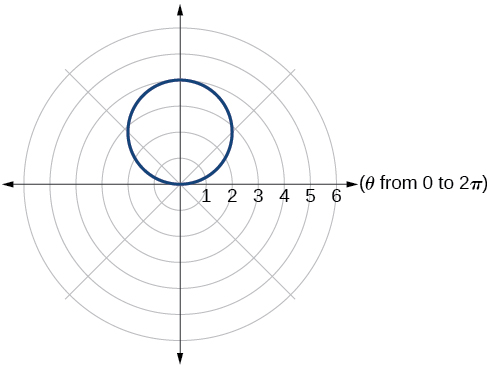$r=2+2\mathrm{cos}\text{\hspace{0.17em}}\theta$

$r=2-2\mathrm{cos}\text{\hspace{0.17em}}\theta$

cardioid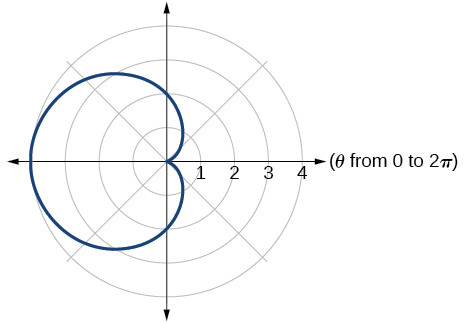$r=5-5\mathrm{sin}\text{\hspace{0.17em}}\theta$

$r=3+3\mathrm{sin}\text{\hspace{0.17em}}\theta$

cardioid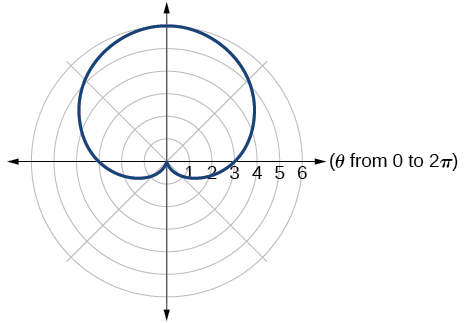$r=3+2\mathrm{sin}\text{\hspace{0.17em}}\theta$

$r=7+4\mathrm{sin}\text{\hspace{0.17em}}\theta$

one-loop/dimpled limaçon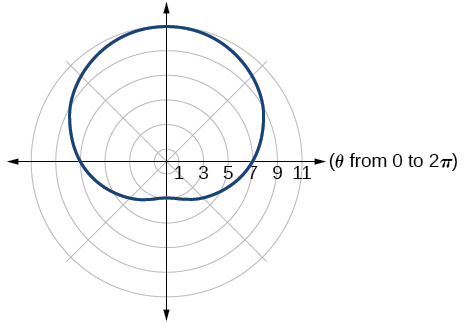$r=4+3\mathrm{cos}\text{\hspace{0.17em}}\theta$

$r=5+4\mathrm{cos}\text{\hspace{0.17em}}\theta$

one-loop/dimpled limaçon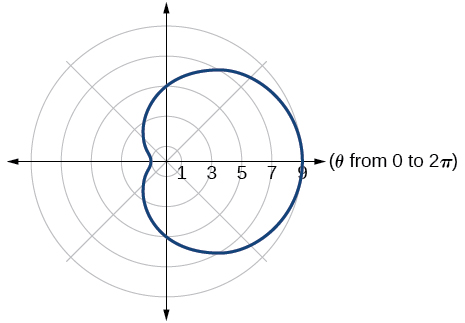$r=10+9\mathrm{cos}\text{\hspace{0.17em}}\theta$

$r=1+3\mathrm{sin}\text{\hspace{0.17em}}\theta$

inner loop/two-loop limaçon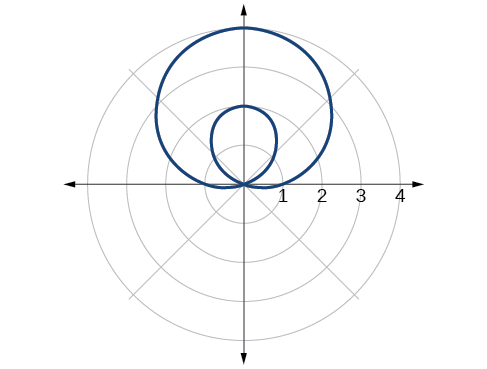$r=2+5\mathrm{sin}\text{\hspace{0.17em}}\theta$

$r=5+7\mathrm{sin}\text{\hspace{0.17em}}\theta$

inner loop/two-loop limaçon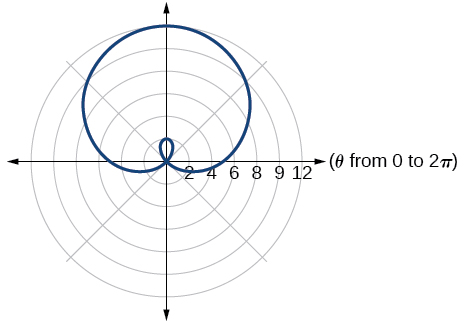$r=2+4\mathrm{cos}\text{\hspace{0.17em}}\theta$

$r=5+6\mathrm{cos}\text{\hspace{0.17em}}\theta$

inner loop/two-loop limaçon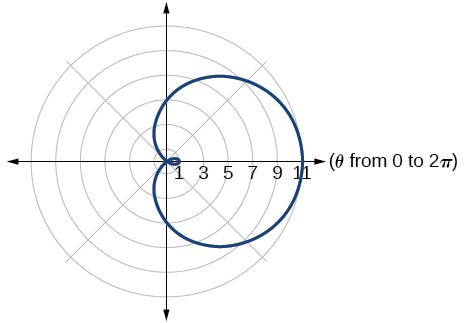${r}^{2}=36\mathrm{cos}\left(2\theta \right)$

${r}^{2}=10\mathrm{cos}\left(2\theta \right)$

lemniscate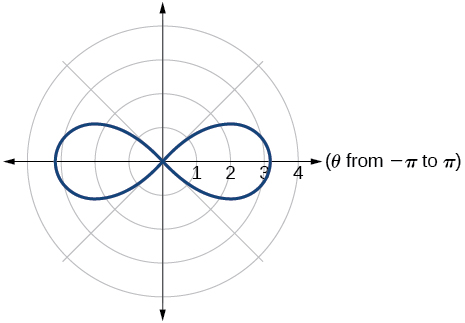${r}^{2}=4\mathrm{sin}\left(2\theta \right)$

${r}^{2}=10\mathrm{sin}\left(2\theta \right)$

lemniscate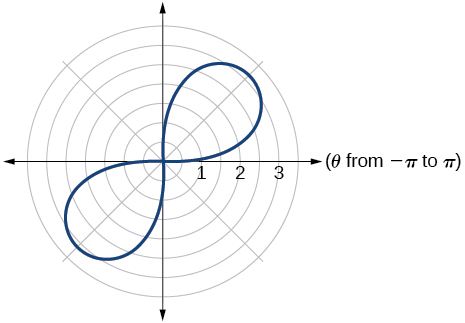$r=3\text{sin}\left(2\theta \right)$

$r=3\text{cos}\left(2\theta \right)$

rose curve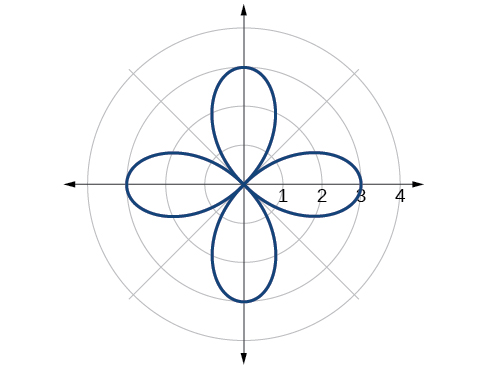$r=5\text{sin}\left(3\theta \right)$

$r=4\text{sin}\left(4\theta \right)$

rose curve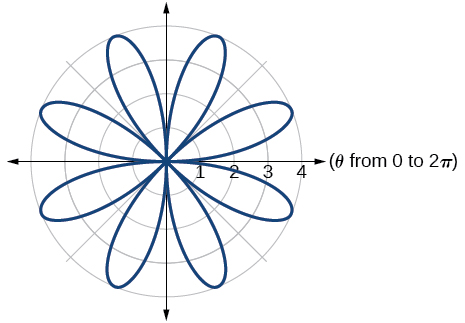$r=4\text{sin}\left(5\theta \right)$

$r=-\theta$

Archimedes’ spiral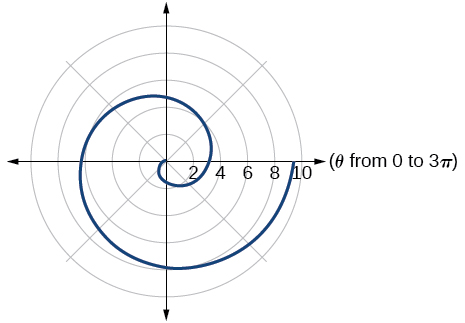$r=2\theta$

$r=-3\theta$

Archimedes’ spiral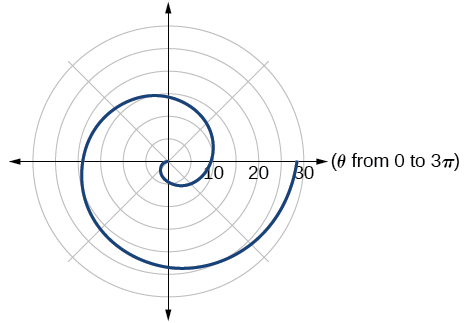## Technology

For the following exercises, use a graphing calculator to sketch the graph of the polar equation.

$r=\frac{1}{\theta }$

$r=\frac{1}{\sqrt{\theta }}$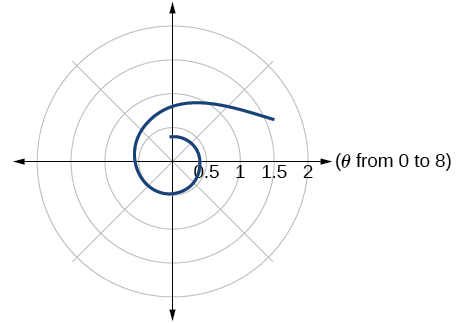$r=2\mathrm{sin}\text{\hspace{0.17em}}\theta \mathrm{tan}\text{\hspace{0.17em}}\theta ,$ a cissoid

$r=2\sqrt{1-{\mathrm{sin}}^{2}\theta }$ , a hippopede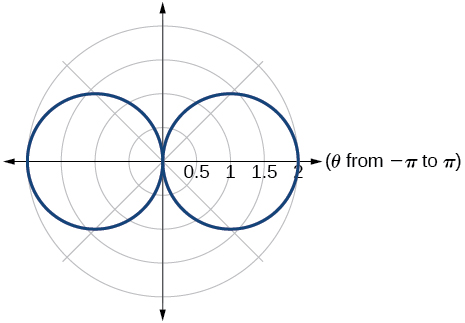$r=5+\mathrm{cos}\left(4\theta \right)$

$r=2-\mathrm{sin}\left(2\theta \right)$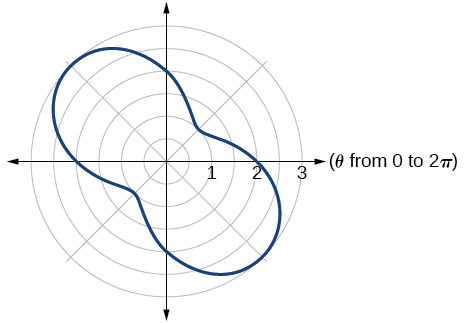$r={\theta }^{2}$

$r=\theta +1$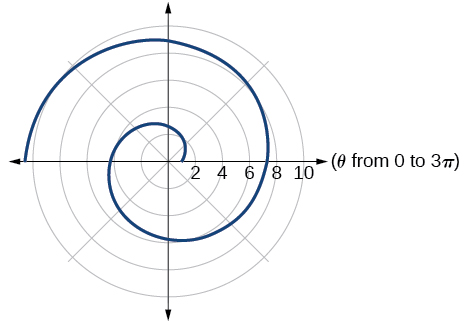$r=\theta \mathrm{sin}\text{\hspace{0.17em}}\theta$

$r=\theta \mathrm{cos}\text{\hspace{0.17em}}\theta$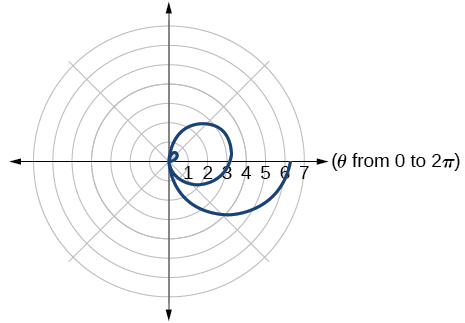For the following exercises, use a graphing utility to graph each pair of polar equations on a domain of $\text{\hspace{0.17em}}\left[0,4\pi \right]\text{\hspace{0.17em}}$ and then explain the differences shown in the graphs.

$r=\theta ,r=-\theta$

$r=\theta ,r=\theta +\mathrm{sin}\text{\hspace{0.17em}}\theta$

They are both spirals, but not quite the same.

$r=\mathrm{sin}\text{\hspace{0.17em}}\theta +\theta ,r=\mathrm{sin}\text{\hspace{0.17em}}\theta -\theta$

$r=2\mathrm{sin}\left(\frac{\theta }{2}\right),r=\theta \mathrm{sin}\left(\frac{\theta }{2}\right)$

Both graphs are curves with 2 loops. The equation with a coefficient of $\text{\hspace{0.17em}}\theta \text{\hspace{0.17em}}$ has two loops on the left, the equation with a coefficient of 2 has two loops side by side. Graph these from 0 to $\text{\hspace{0.17em}}4\pi \text{\hspace{0.17em}}$ to get a better picture.

$r=\mathrm{sin}\left(\mathrm{cos}\left(3\theta \right)\right)\text{\hspace{0.17em}}\text{\hspace{0.17em}}r=\mathrm{sin}\left(3\theta \right)$

On a graphing utility, graph $\text{\hspace{0.17em}}r=\mathrm{sin}\left(\frac{16}{5}\theta \right)\text{\hspace{0.17em}}$ on $\text{\hspace{0.17em}}\left[0,4\pi \right],\left[0,8\pi \right],\left[0,12\pi \right],\text{\hspace{0.17em}}$ and $\text{\hspace{0.17em}}\left[0,16\pi \right].\text{\hspace{0.17em}}$ Describe the effect of increasing the width of the domain.

When the width of the domain is increased, more petals of the flower are visible.

On a graphing utility, graph and sketch $\text{\hspace{0.17em}}r=\mathrm{sin}\text{\hspace{0.17em}}\theta +{\left(\mathrm{sin}\left(\frac{5}{2}\theta \right)\right)}^{3}\text{\hspace{0.17em}}$ on $\text{\hspace{0.17em}}\left[0,4\pi \right].$

On a graphing utility, graph each polar equation. Explain the similarities and differences you observe in the graphs.

$\begin{array}{l}\begin{array}{l}\\ {r}_{1}=3\mathrm{sin}\left(3\theta \right)\end{array}\hfill \\ {r}_{2}=2\mathrm{sin}\left(3\theta \right)\hfill \\ {r}_{3}=\mathrm{sin}\left(3\theta \right)\hfill \end{array}$

The graphs are three-petal, rose curves. The larger the coefficient, the greater the curve’s distance from the pole.

On a graphing utility, graph each polar equation. Explain the similarities and differences you observe in the graphs.

$\begin{array}{l}\begin{array}{l}\\ {r}_{1}=3+3\mathrm{cos}\text{\hspace{0.17em}}\theta \end{array}\hfill \\ {r}_{2}=2+2\mathrm{cos}\text{\hspace{0.17em}}\theta \hfill \\ {r}_{3}=1+\mathrm{cos}\text{\hspace{0.17em}}\theta \hfill \end{array}$

On a graphing utility, graph each polar equation. Explain the similarities and differences you observe in the graphs.

$\begin{array}{l}\begin{array}{l}\\ {r}_{1}=3\theta \end{array}\hfill \\ {r}_{2}=2\theta \hfill \\ {r}_{3}=\theta \hfill \end{array}$

The graphs are spirals. The smaller the coefficient, the tighter the spiral.

## Extensions

For the following exercises, draw each polar equation on the same set of polar axes, and find the points of intersection.

${r}_{1}=3+2\mathrm{sin}\text{\hspace{0.17em}}\theta ,\text{\hspace{0.17em}}{r}_{2}=2$

${r}_{1}=6-4\mathrm{cos}\text{\hspace{0.17em}}\theta ,\text{\hspace{0.17em}}{r}_{2}=4$

$\left(4,\frac{\pi }{3}\right),\left(4,\frac{5\pi }{3}\right)$

${r}_{1}=1+\mathrm{sin}\text{\hspace{0.17em}}\theta ,\text{\hspace{0.17em}}{r}_{2}=3\mathrm{sin}\text{\hspace{0.17em}}\theta$

${r}_{1}=1+\mathrm{cos}\text{\hspace{0.17em}}\theta ,\text{\hspace{0.17em}}{r}_{2}=3\mathrm{cos}\text{\hspace{0.17em}}\theta$

$\left(\frac{3}{2},\frac{\pi }{3}\right),\left(\frac{3}{2},\frac{5\pi }{3}\right)$

${r}_{1}=\mathrm{cos}\left(2\theta \right),\text{\hspace{0.17em}}{r}_{2}=\mathrm{sin}\left(2\theta \right)$

${r}_{1}={\mathrm{sin}}^{2}\left(2\theta \right),\text{\hspace{0.17em}}{r}_{2}=1-\mathrm{cos}\left(4\theta \right)$

$\left(0,\frac{\pi }{2}\right),\text{\hspace{0.17em}}\left(0,\pi \right),\text{\hspace{0.17em}}\left(0,\frac{3\pi }{2}\right),\text{\hspace{0.17em}}\left(0,2\pi \right)$

${r}_{1}=\sqrt{3},\text{\hspace{0.17em}}{r}_{2}=2\mathrm{sin}\left(\theta \right)$

${r}_{1}{}^{2}=\mathrm{sin}\text{\hspace{0.17em}}\theta ,{r}_{2}{}^{2}=\mathrm{cos}\text{\hspace{0.17em}}\theta$

$\left(\frac{\sqrt{8}}{2},\frac{\pi }{4}\right),\text{\hspace{0.17em}}\left(\frac{\sqrt{8}}{2},\frac{5\pi }{4}\right)\text{\hspace{0.17em}}$ and at $\text{\hspace{0.17em}}\theta =\frac{3\pi }{4},\text{\hspace{0.17em}}\text{\hspace{0.17em}}\frac{7\pi }{4}\text{\hspace{0.17em}}\text{\hspace{0.17em}}$ since $\text{\hspace{0.17em}}r\text{\hspace{0.17em}}$ is squared

${r}_{1}=1+\mathrm{cos}\text{\hspace{0.17em}}\theta ,\text{\hspace{0.17em}}{r}_{2}=1-\mathrm{sin}\text{\hspace{0.17em}}\theta$

what are you up to?
nothing up todat yet
Miranda
hi
jai
hello
jai
Miranda Drice
jai
aap konsi country se ho
jai
which language is that
Miranda
I am living in india
jai
good
Miranda
what is the formula for calculating algebraic
I think the formula for calculating algebraic is the statement of the equality of two expression stimulate by a set of addition, multiplication, soustraction, division, raising to a power and extraction of Root. U believe by having those in the equation you will be in measure to calculate it
Miranda
state and prove Cayley hamilton therom
hello
Propessor
hi
Miranda
the Cayley hamilton Theorem state if A is a square matrix and if f(x) is its characterics polynomial then f(x)=0 in another ways evey square matrix is a root of its chatacteristics polynomial.
Miranda
hi
jai
hi Miranda
jai
thanks
Propessor
welcome
jai
What is algebra
algebra is a branch of the mathematics to calculate expressions follow.
Miranda
Miranda Drice would you mind teaching me mathematics? I think you are really good at math. I'm not good at it. In fact I hate it. 😅😅😅
Jeffrey
lolll who told you I'm good at it
Miranda
something seems to wispher me to my ear that u are good at it. lol
Jeffrey
lolllll if you say so
Miranda
but seriously, Im really bad at math. And I hate it. But you see, I downloaded this app two months ago hoping to master it.
Jeffrey
which grade are you in though
Miranda
oh woww I understand
Miranda
Jeffrey
Jeffrey
Miranda
how come you finished in college and you don't like math though
Miranda
gotta practice, holmie
Steve
if you never use it you won't be able to appreciate it
Steve
I don't know why. But Im trying to like it.
Jeffrey
yes steve. you're right
Jeffrey
so you better
Miranda
what is the solution of the given equation?
which equation
Miranda
I dont know. lol
Jeffrey
Miranda
Jeffrey
answer and questions in exercise 11.2 sums
how do u calculate inequality of irrational number?
Alaba
give me an example
Chris
and I will walk you through it
Chris
cos (-z)= cos z .
cos(- z)=cos z
Mustafa
what is a algebra
(x+x)3=?
6x
Obed
what is the identity of 1-cos²5x equal to?
__john __05
Kishu
Hi
Abdel
hi
Ye
hi
Nokwanda
C'est comment
Abdel
Hi
Amanda
hello
SORIE
Hiiii
Chinni
hello
Ranjay
hi
ANSHU
hiiii
Chinni
h r u friends
Chinni
yes
Hassan
so is their any Genius in mathematics here let chat guys and get to know each other's
SORIE
I speak French
Abdel
okay no problem since we gather here and get to know each other
SORIE
hi im stupid at math and just wanna join here
Yaona
lol nahhh none of us here are stupid it's just that we have Fast, Medium, and slow learner bro but we all going to work things out together
SORIE
it's 12
what is the function of sine with respect of cosine , graphically
tangent bruh
Steve
cosx.cos2x.cos4x.cos8x
sinx sin2x is linearly dependent
what is a reciprocal
The reciprocal of a number is 1 divided by a number. eg the reciprocal of 10 is 1/10 which is 0.1
Shemmy
Reciprocal is a pair of numbers that, when multiplied together, equal to 1. Example; the reciprocal of 3 is ⅓, because 3 multiplied by ⅓ is equal to 1
Jeza
each term in a sequence below is five times the previous term what is the eighth term in the sequence
I don't understand how radicals works pls
How look for the general solution of a trig functionByByBy OpenStaxByBy Anh DaoByBy Jesenia WoffordBy Danielle StephensBy Angela JanuaryBy Madison ChristianBy Madison ChristianBy OpenStax